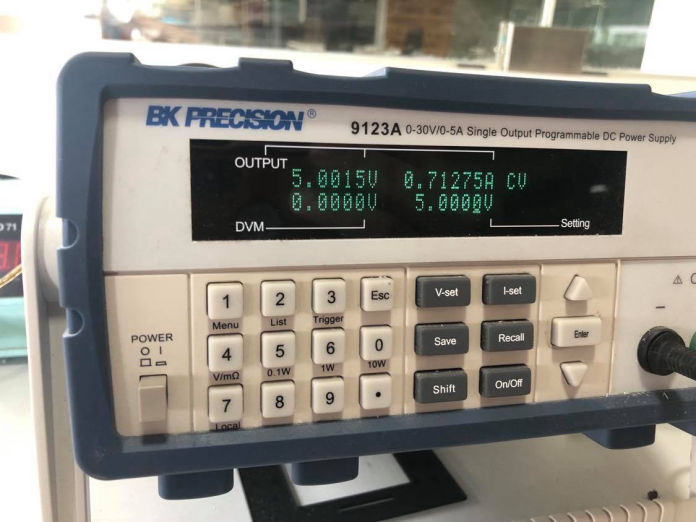## 12.Output Devices:

### 1. Objectives:

2.Group Assignment: measure the power consumption of an output device

3.Individual Assignment:

• add an output device to a microcontroller board you've designed
• and program it to do something

### 4. Files, Planning, Tools:

Files:

Planning:
• See how to best use this week to help complete the final project, as well.
• Design Servo Enclosure[For final project].
• Design a minimalist PCB to run a servo motor
• See how to connect two distinct, disconnected PCBs

Tools:

• Fusion 360
• Autodesk Eagle
• OnShape

1. I was away from Lab this week, too, due to covid uncertanities
2. Of all the weeks that will help me complete my final project, this is the most directly connected. Final Project: A Home Automation project that actuates household switches with a servo motor.
4. This is by far the best article I have read on the topic - here
5. ## Servo Motor:

6. In the most generic sense, a "servomechanism" (servo for short) is a device that uses feedback to achieve the desired result.
7.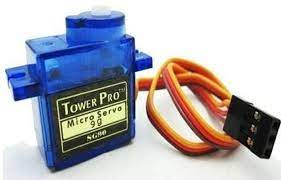8. RC servos are reasonably standardized - they are all a similar shape, with mounting flanges at each end, available in graduated sizes. Servos often come with several wheels or levers, known as "horns", than can be attached to the shaft, to fit the device they are operating.
9.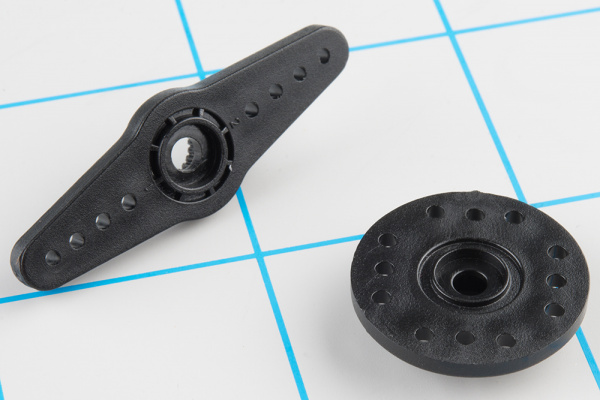10. servos use a standard type of 3-pin plug
11. Control Signal:Servos are controlled with a specific type of pulse train signal. The pulses occur at a 20 mSec (50 Hz) interval, and vary between 1 and 2 mSec in width. The Pulse Width Modulation hardware available on a microcontroller is a great way to generate servo control signals. Common servos rotate over a range of 90° as the pulses vary between 1 and 2 mSec -- they should be at the center of their mechanical range when the pulse is 1.5 mSec.
12. Internally, the mechanism of a servo motor uses a potentiometer attached to the rotating shaft to sense the position. It measures the width of the incoming pulse, and applies current to the motor to turn the shaft correspondingly.
13.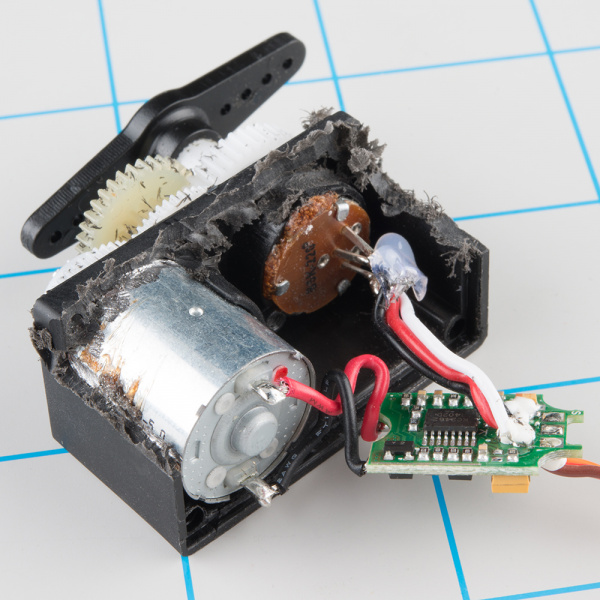Credit:here
14. The other side of the PCB has some transistors in an H-bridge configuration, which allows the controller to steer current through the motor in either direction, for both clockwise and counterclockwise rotation.
15. Ordinary RC servos turn over a 90° range -- it's useful for turning a steering linkage, or adjusting the control surfaces on an airplane, but not so useful as a drive mechanism. That's where full or continuous rotation servos come in. But Since I need very limited range for my final project, I have no use for it.
16. Started going through the ATTiny85 Datasheet for timer - here
17. This YT Video helped a lot, so did this Electronics Stackexchange Q.
18. After a long discussion with my friend Saheen, I have decided that I will use ATTiny412 1-Series MC.
19. Then started a 6hrs+ read of various articles, documentations, datsheets, video transcripts, previous year documentations...
20. Here are few important links:
21.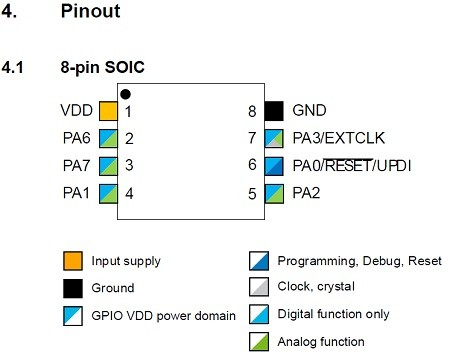22. Rough initial ATTiny412 sketch for step_response input, and servo motor output, done in EasyEDA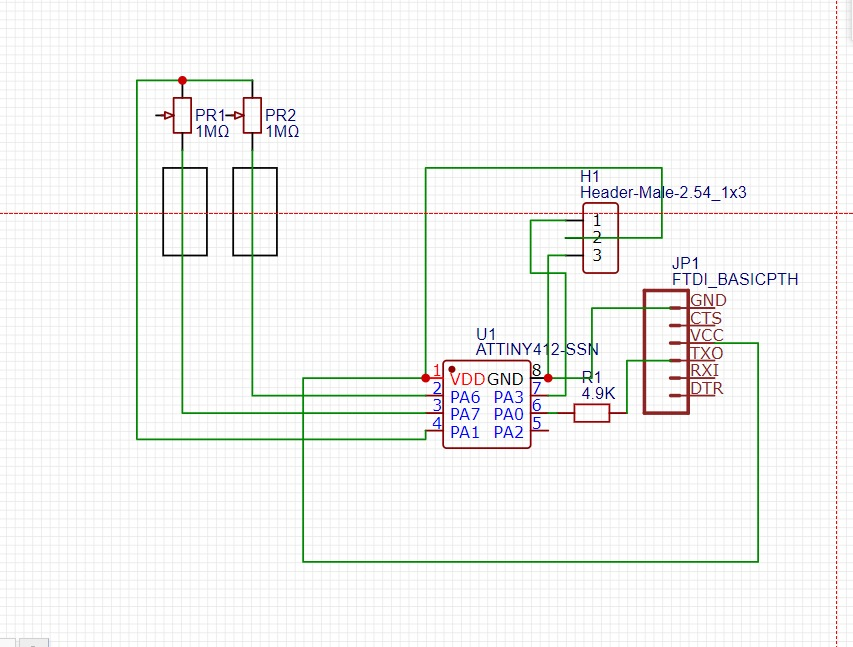23. I was told that we cannot program as well as power through same FTDI. Then, Adrian recommended me to UDPI+VCC module
24.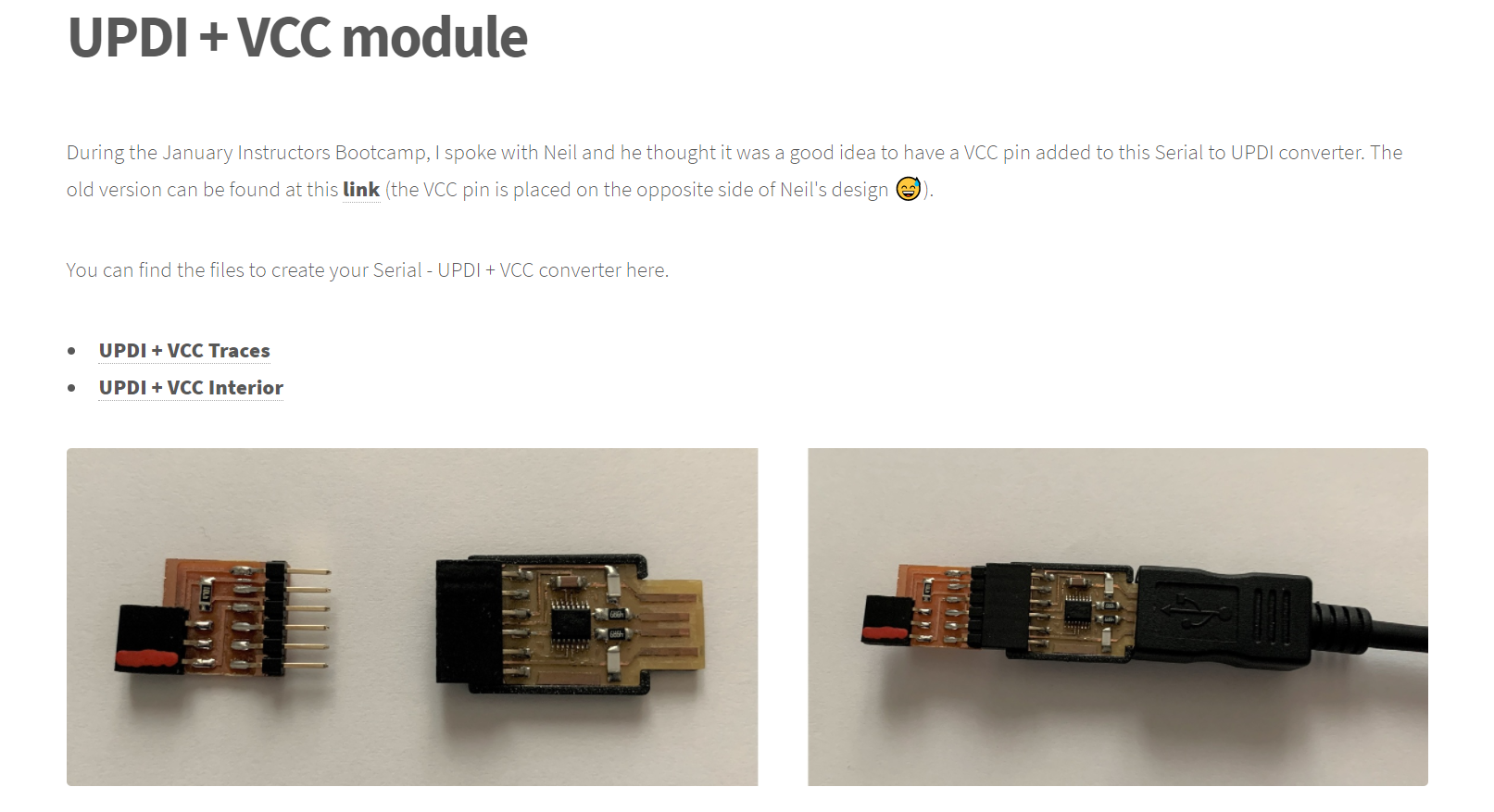25. I realized that circuit design is not very intutitve to me, currently. I have spent an entire day surfing internet.
26. To simplify the matters a bit, I have reverted back to ATTiny85
27. Components Used:
• Voltage Regulator {Initially, I used a voltage divider with two resistors}
• ATTiny85
• Resistor for Reset PIN
• Capacitor after voltage regulator for steady flow
28. Initial schematic:
29.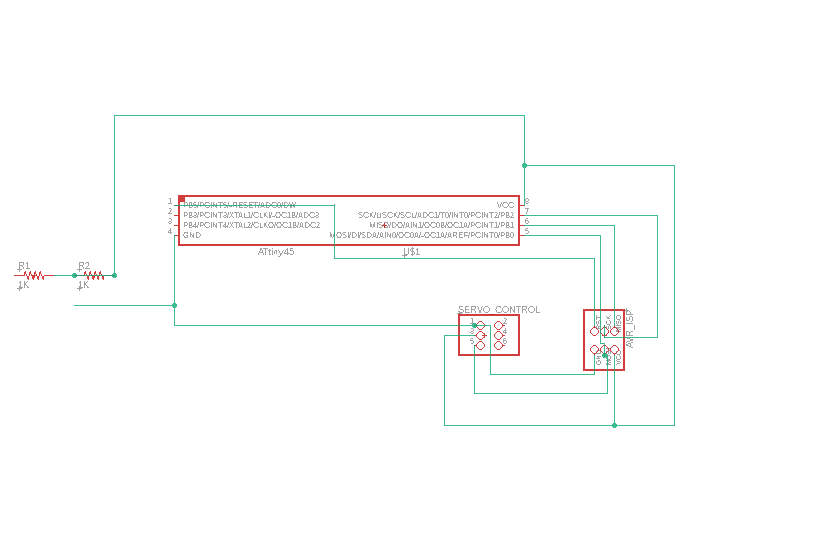30. Updated Schematic:
31.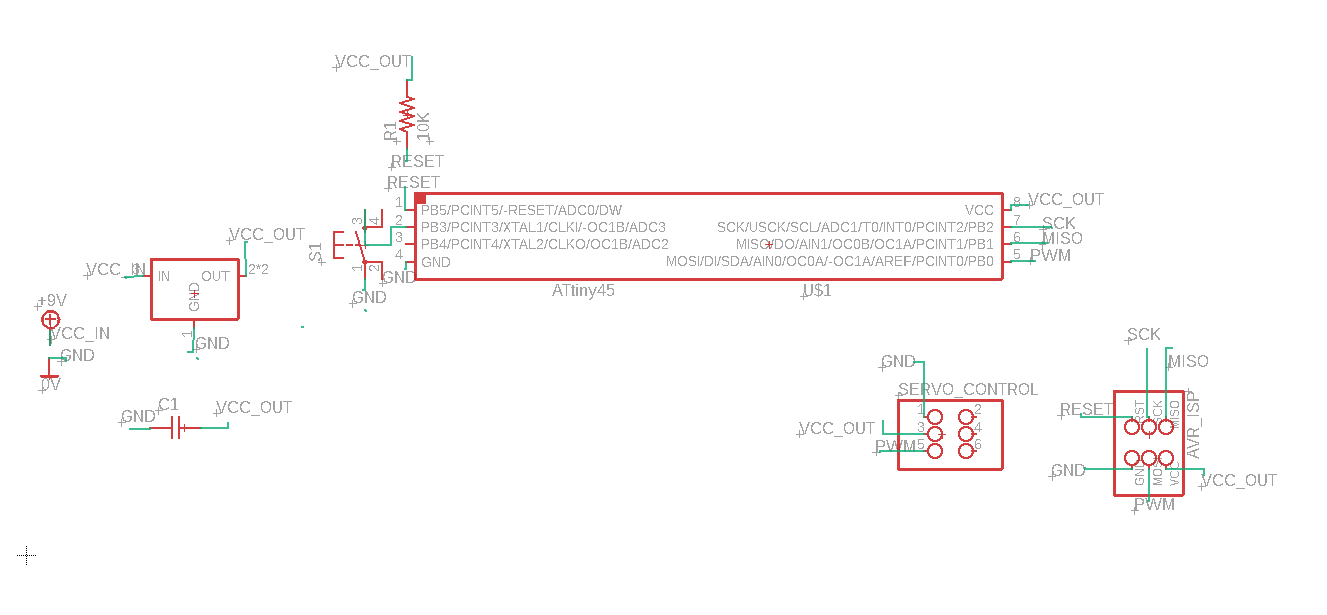32. The Board:
33.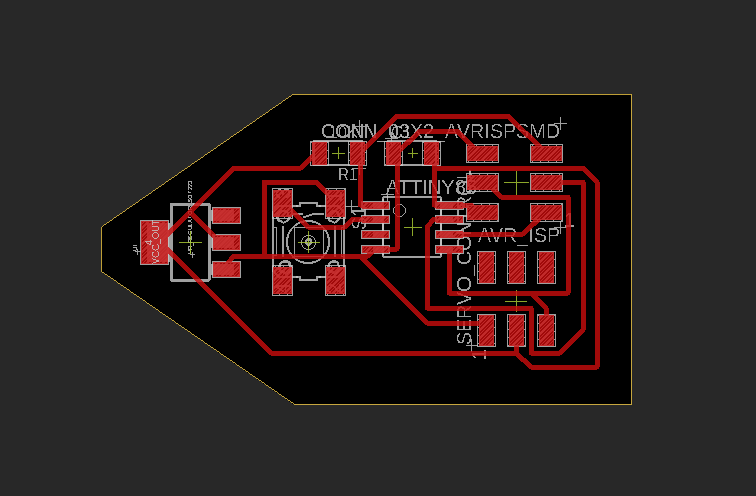34. The Board in Monochrome:
35.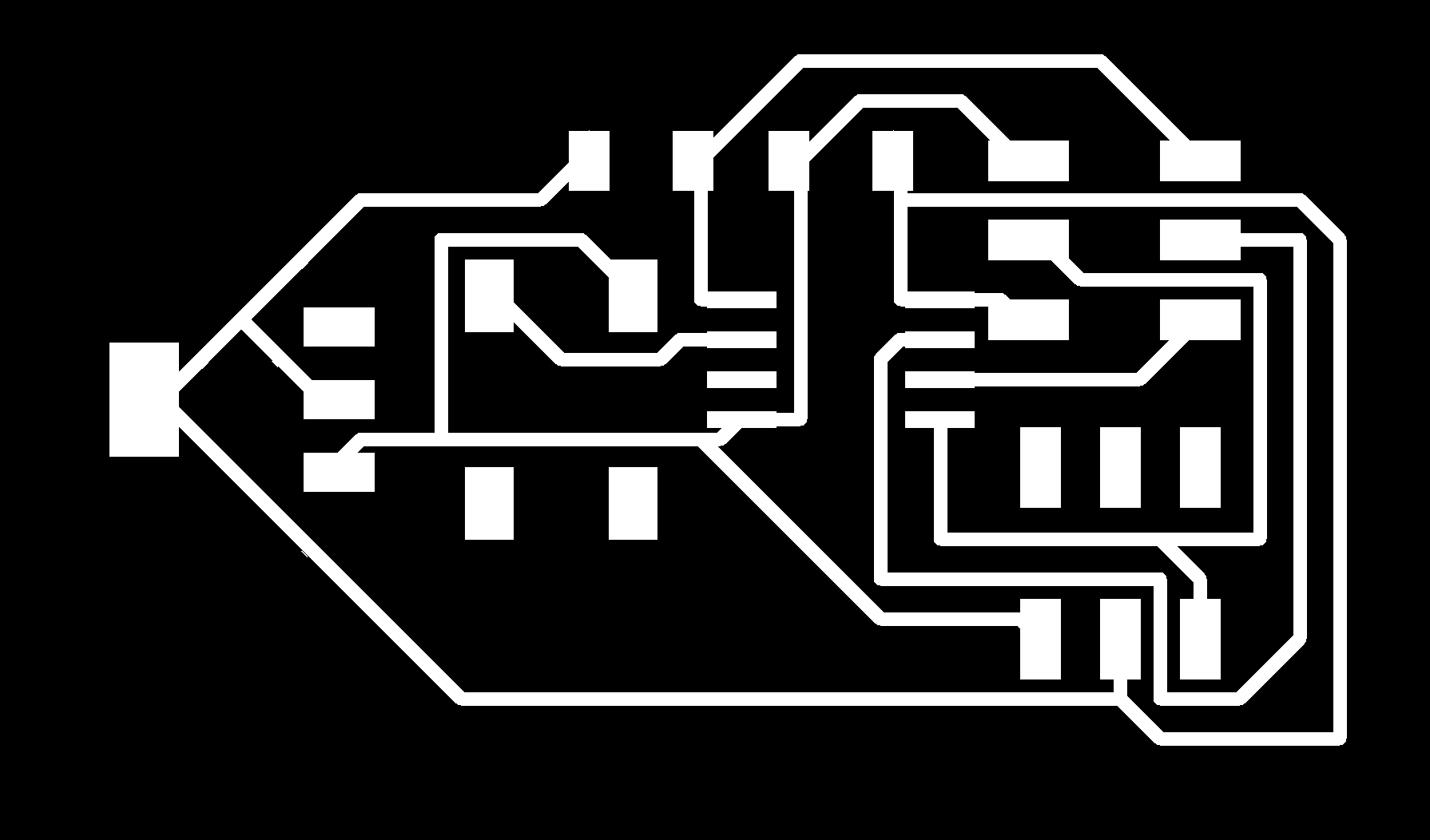```#include Servo.h
Servo myservo;
int pos = 0;

void setup() {

myservo.attach(0);  // PWM pin PB0

}

void loop() {
for (pos = 0; pos <= 160; pos += 2)
{
myservo.write(pos);
delay(80);
}

for (pos = 160; pos >= 0; pos -= 2)
{
myservo.write(pos);
delay(80);
}
}
```
36. We are currently in a lockdown, and I will get back to fabricating it, once I reach the lab. Thanks.

### After Reaching The Lab:

1. #### Schematic: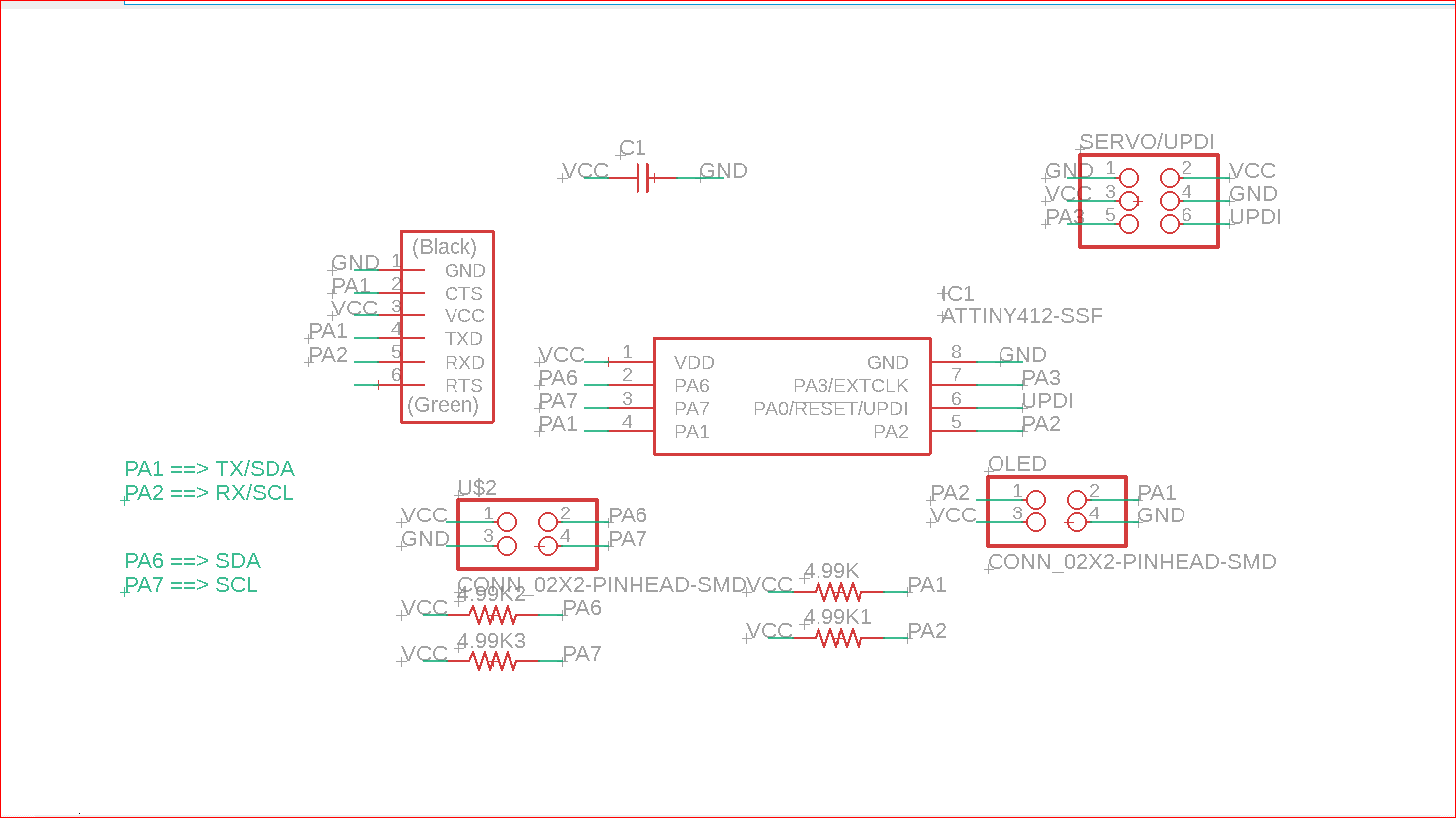2. #### Reasoning: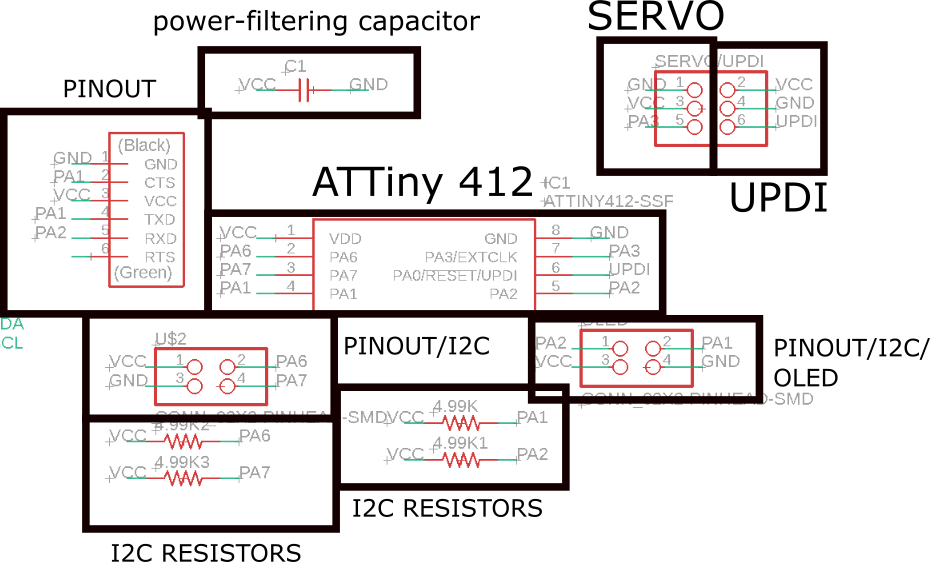3. #### Board: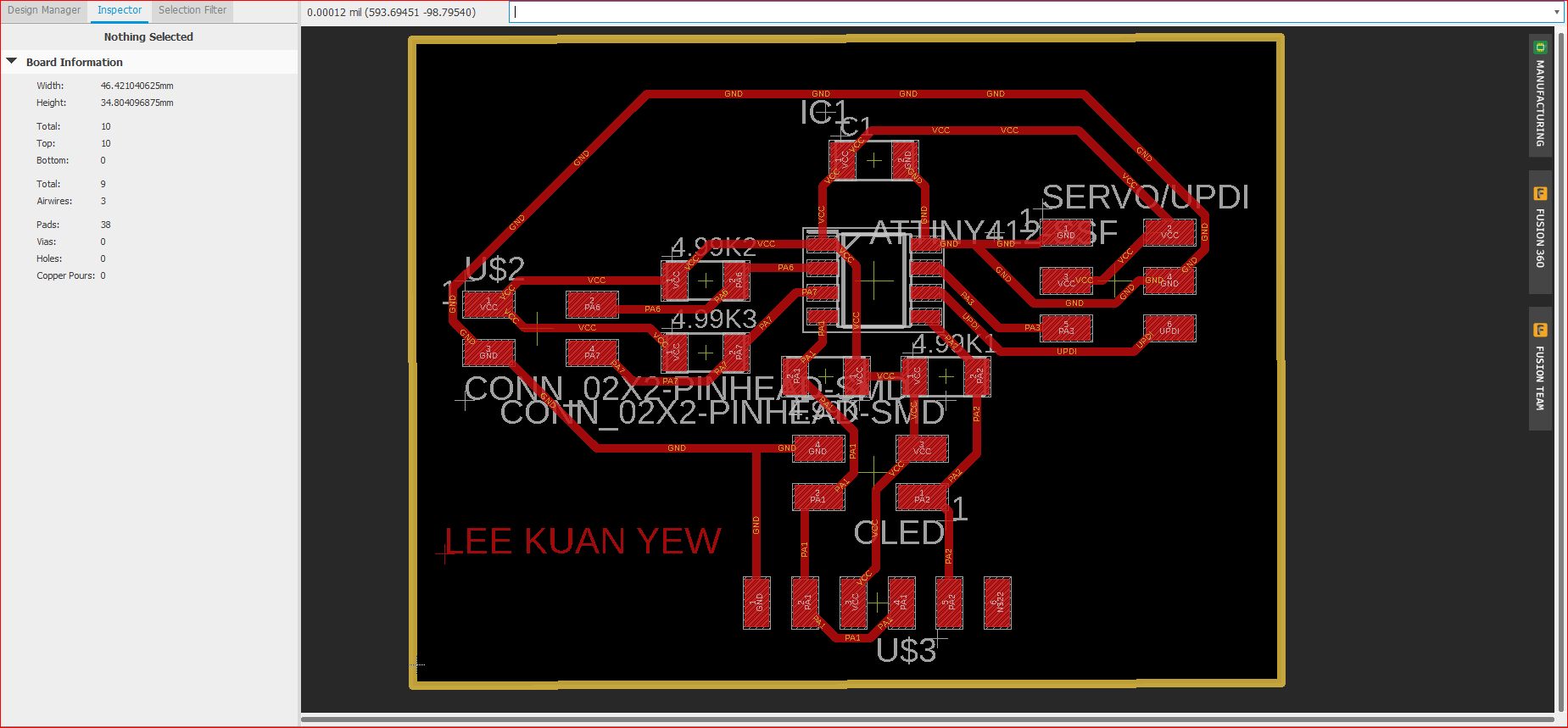4. #### Trivial ERC Errors: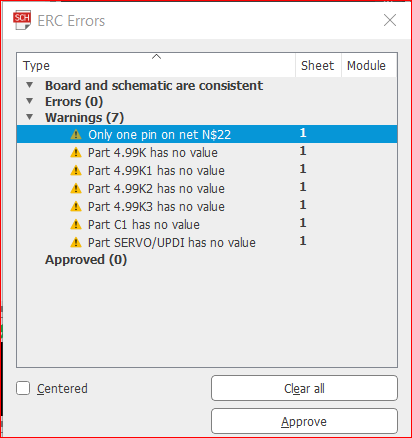5. #### DRC: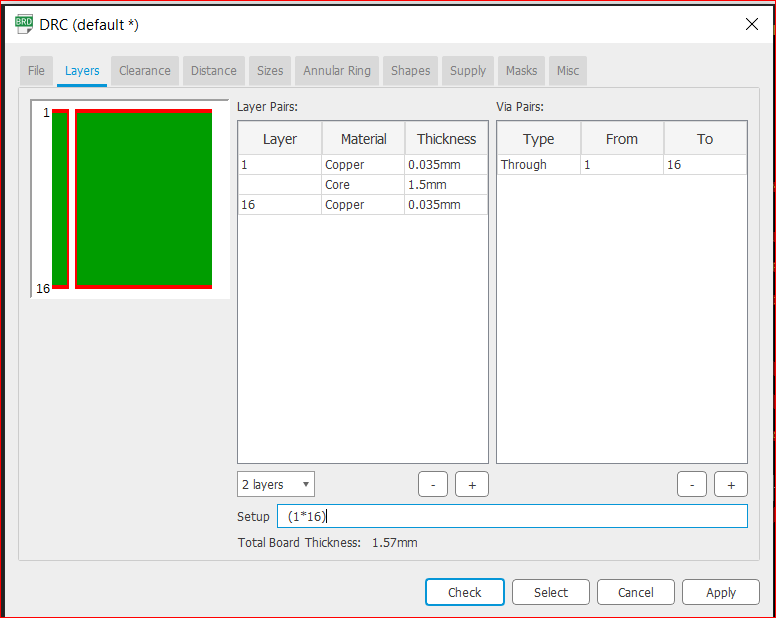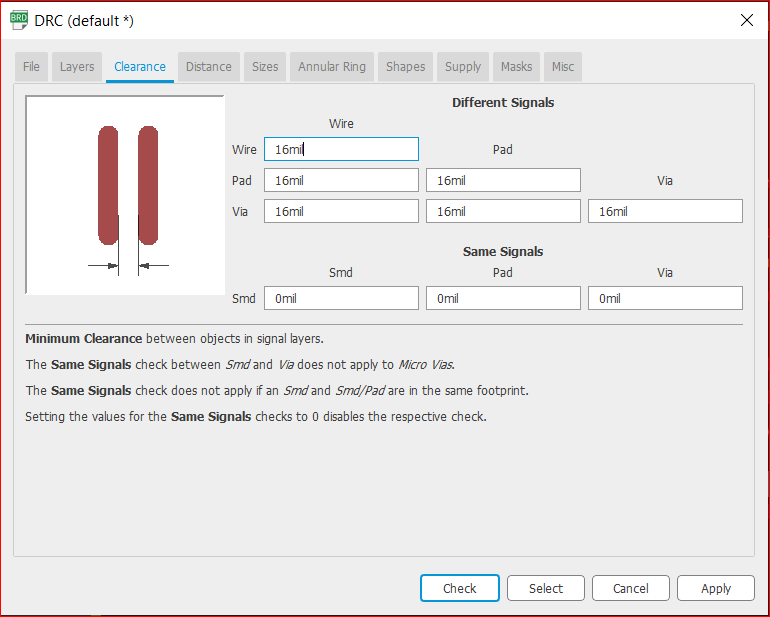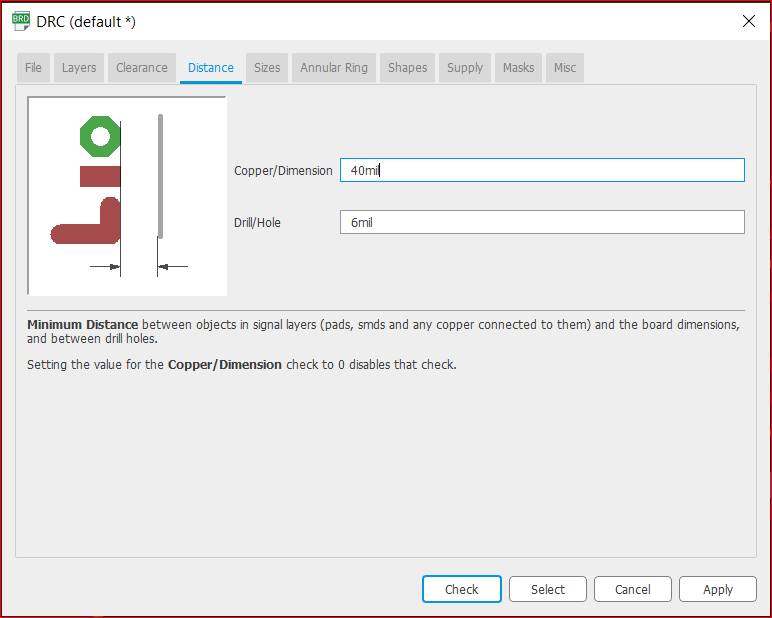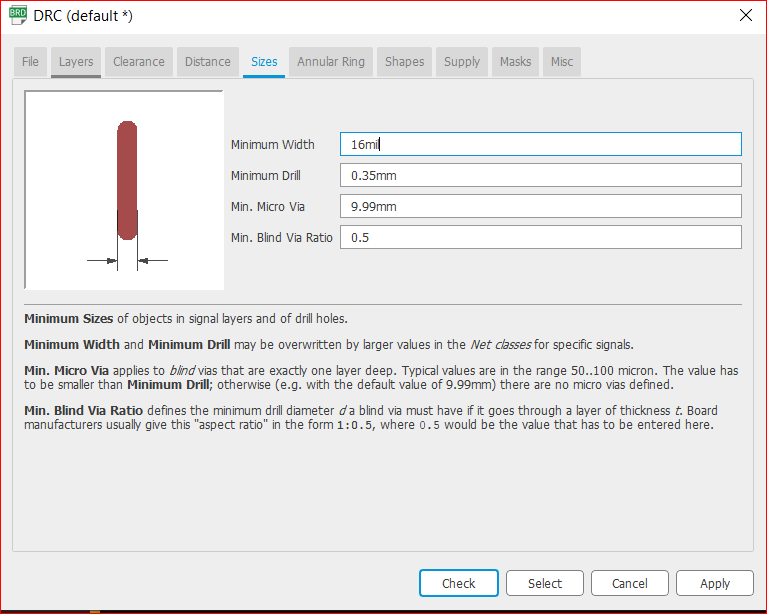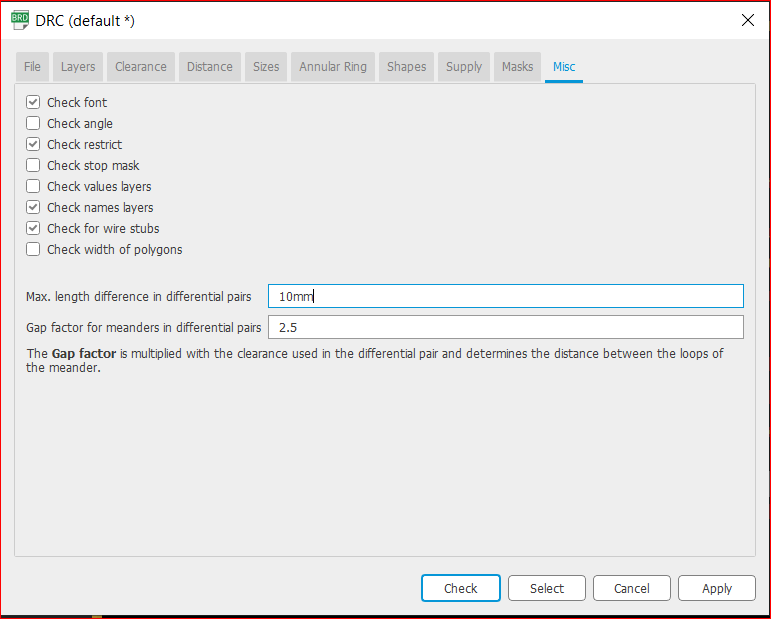6. #### Image for Reference: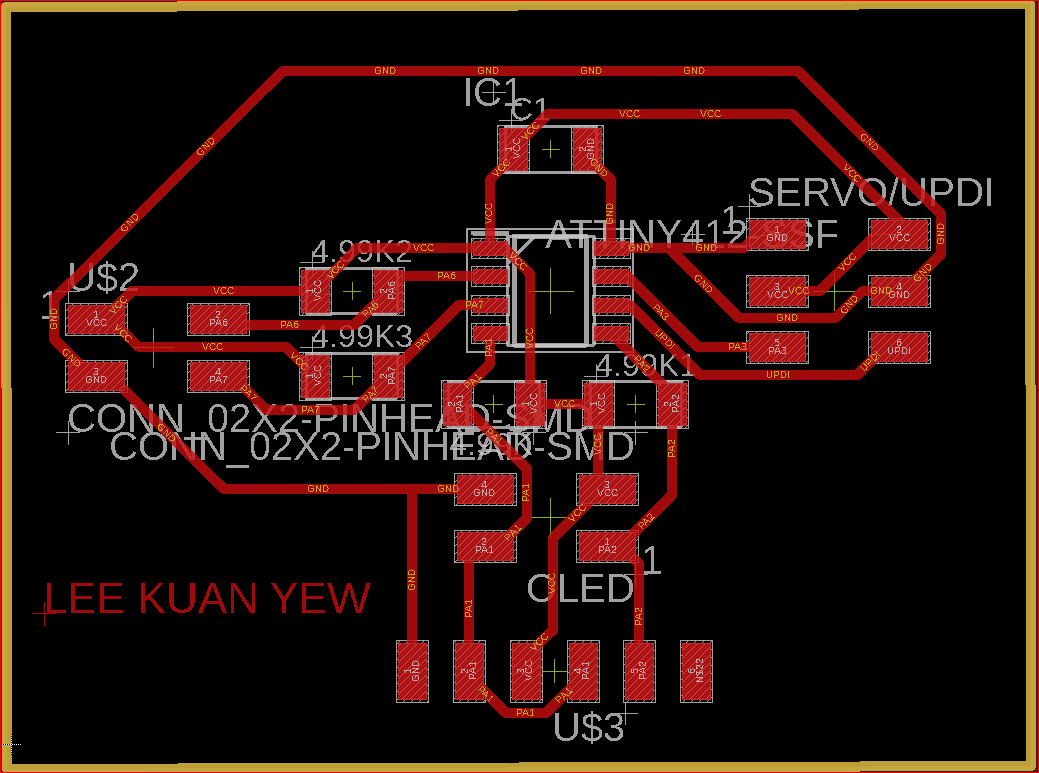7. #### Traces: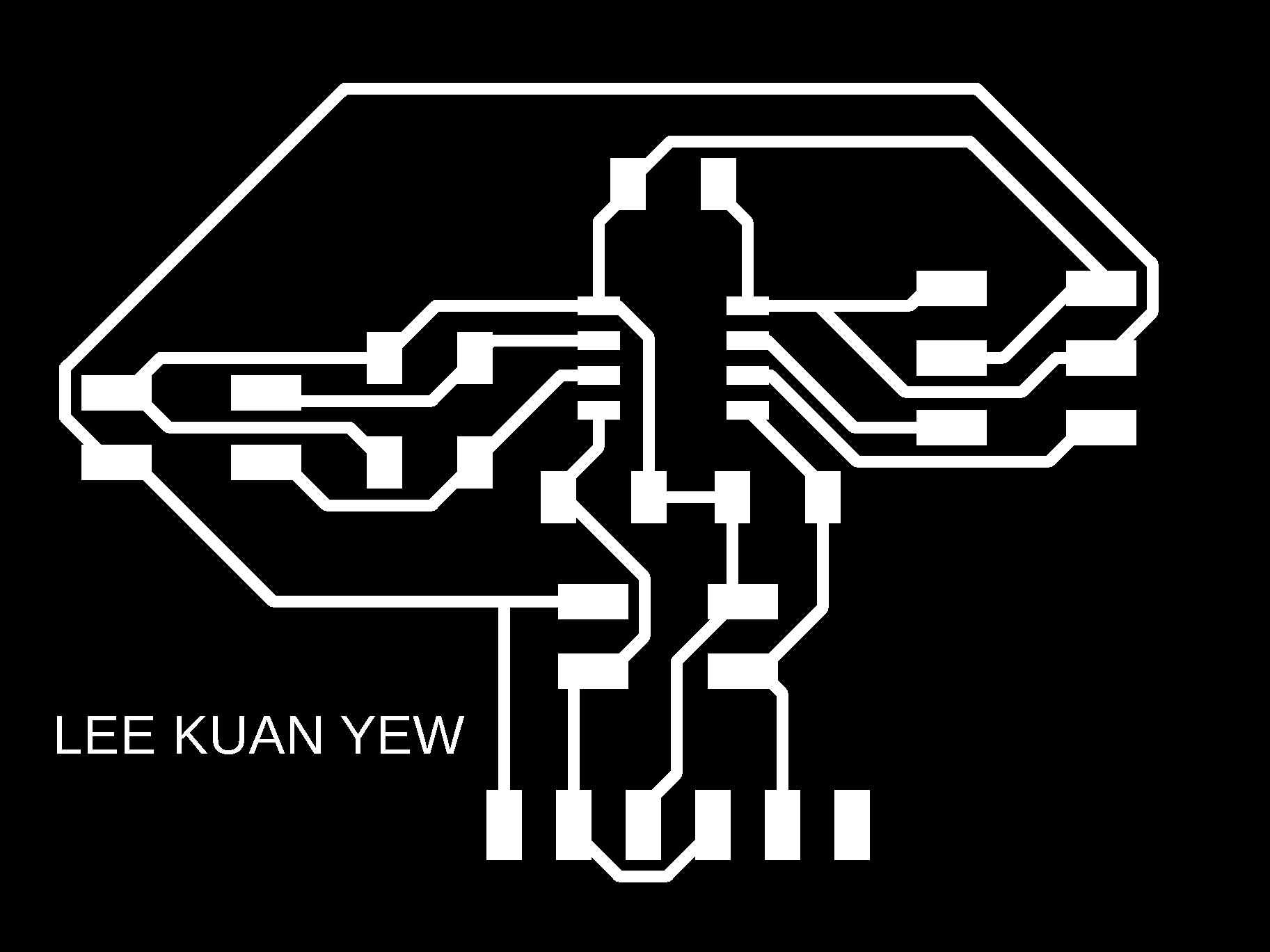8. #### Outline:9. #### Fabrication: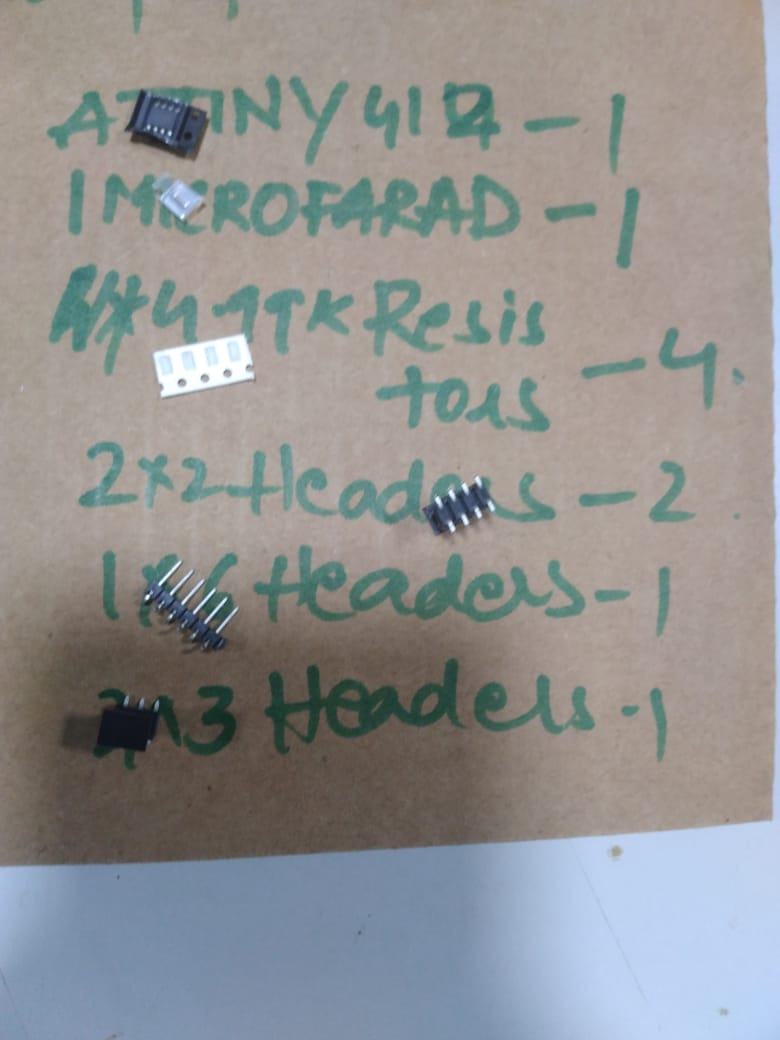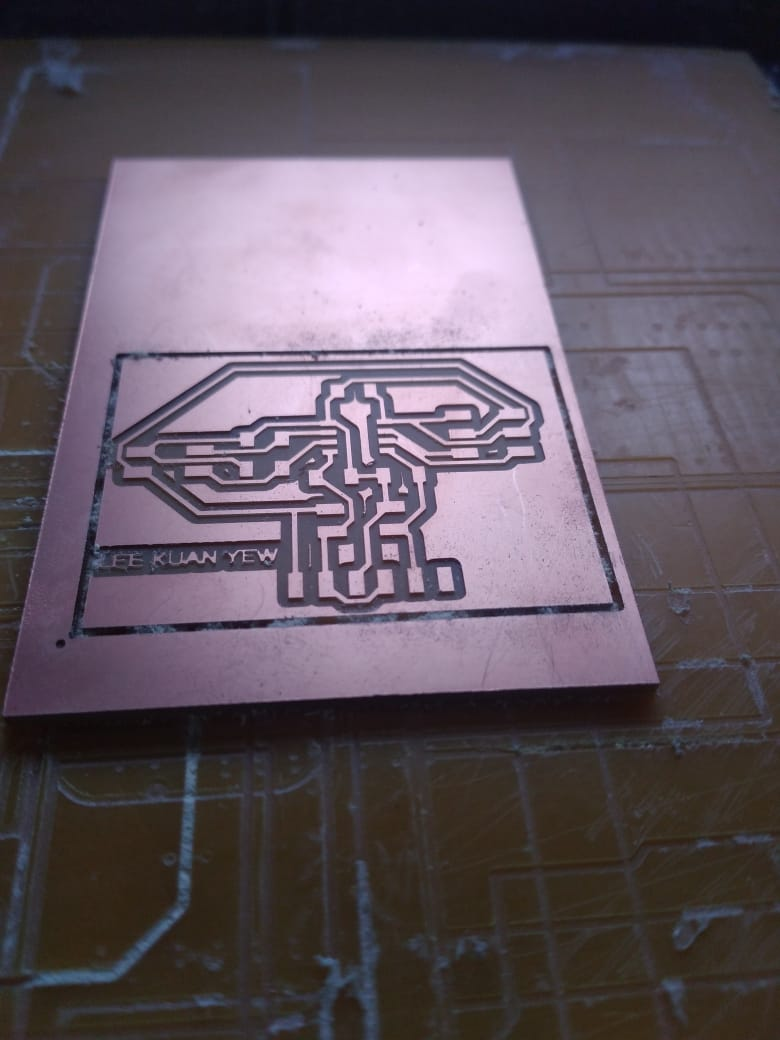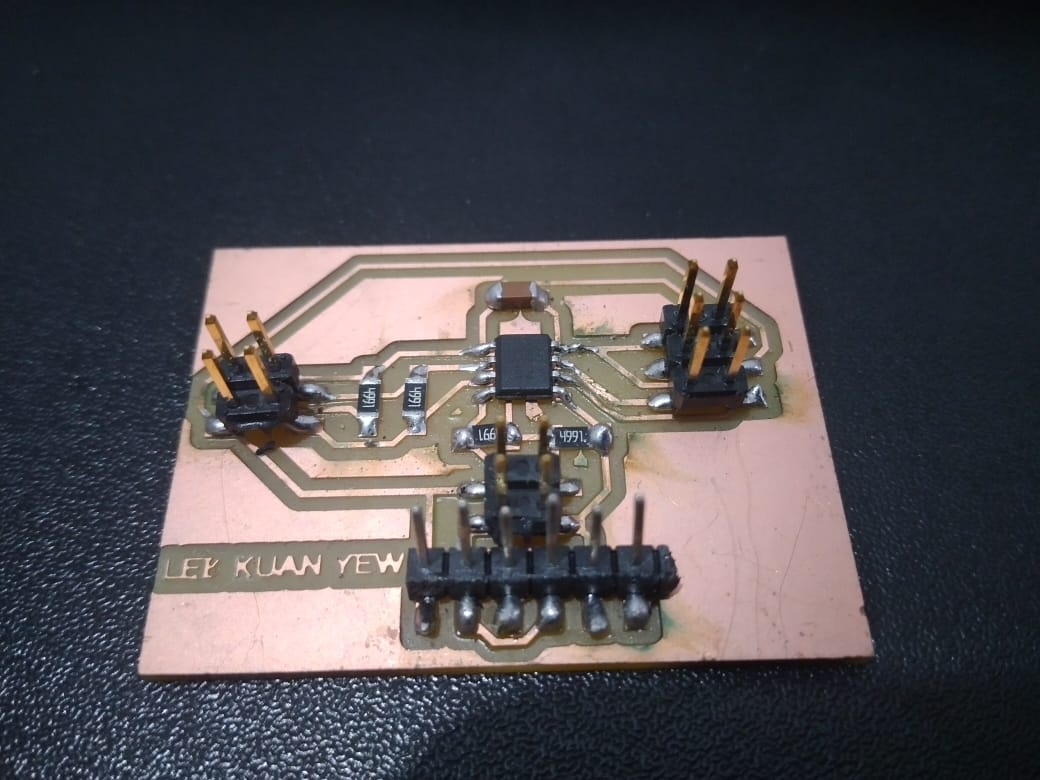10. #### Programming:

I programmed my microntroller using UPDI pin, with the help of megaTinyCore library, and I used UPDI + VCC module.

1. Servo
2. LCD
12. #### Servo:

To control a servo, we need a GND, VCC, and a Analog Pin. Maximum Voltage of my servo is 6v.

```          ```
#include

Servo servo;

int pos = 0;
int pin = 1;

void setup() {
servo.attach(pin);
}

void loop() {
for (pos = 0; pos <= 180; pos += 1) {
// in steps of 1 degree
servo.write(pos);
delay(15);
}
for (pos = 180; pos >= 0; pos -= 1) {
servo.write(pos);
delay(15);
}
}
```
```
Connect Servo Pin to PA7 Pin, which maps to Arduino "1".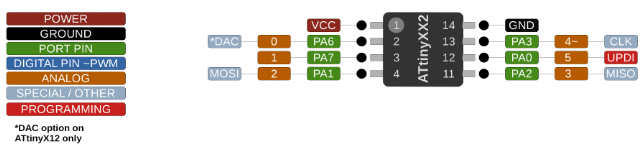13. #### LCD:

The LCD 2004A can be controlled by either I2C or SPI, I chose I2C. Therefore, we need 2 more pins apart from SCL and SDA. LCD 2004A needs 5v-10v. It has 4 rows, and 20 columns.

```          ```
#include  // Library for I2C communication
#include  // Library for LCD

LiquidCrystal_I2C lcd(0x27, 20, 4);
void setup() {
Wire.begin();
lcd.init();
lcd.backlight();
}

void loop() {
lcd.clear();
lcd.home();
lcd.print("Regression");
lcd.setCursor(2, 1);
lcd.print("To The Mean");
lcd.setCursor(0, 2);
lcd.setCursor(9 ,3);
lcd.print("->Jai Hanani");
delay(200);

}
```
```
Native Arduino LiquidCrystal Library, and there you can find the documentation for all the commands I used in the code.
14. ### Group Assignment:

For the group assignment, we used our colleague's output devices board to measure its power. The following code was used to see the difference in current and then calculate the power consumption of both the connected servo and buzzer.

```          ```
#include
#define buzz 4
Servo myservo;

int angle = 0;

void setup() {
pinMode(buzz, OUTPUT);
myservo.attach(0);

}

void loop() {
for (angle = 0; angle <= 180; angle += 1) {
myservo.write(angle);
delay(20);
}
delay(100);
for ( angle = 180; angle >= 0; angle -= 1) {
myservo.write(angle);
delay(20);

}
digitalWrite(buzz, 1);
delay(2000);
digitalWrite(buzz, 0);
delay(2000);
}
```
```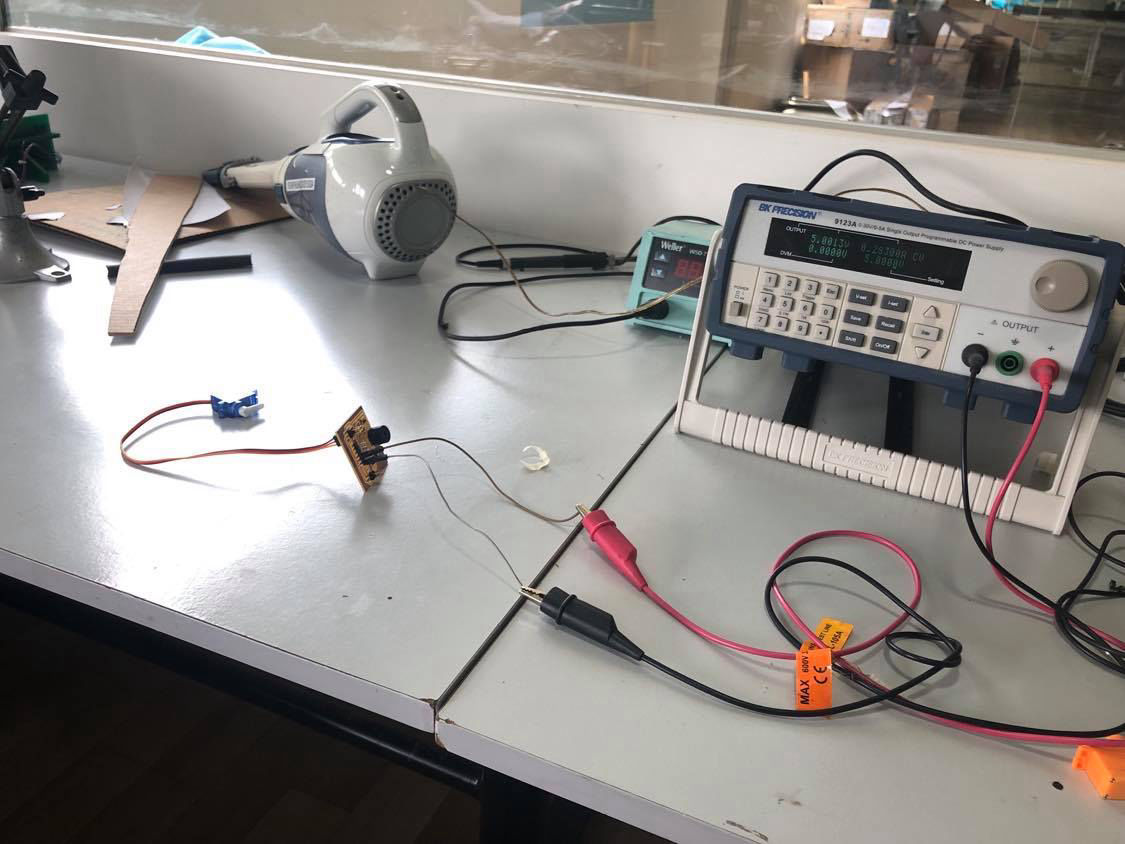No Output Connected ==> Voltage = 5V; Current = 0.011A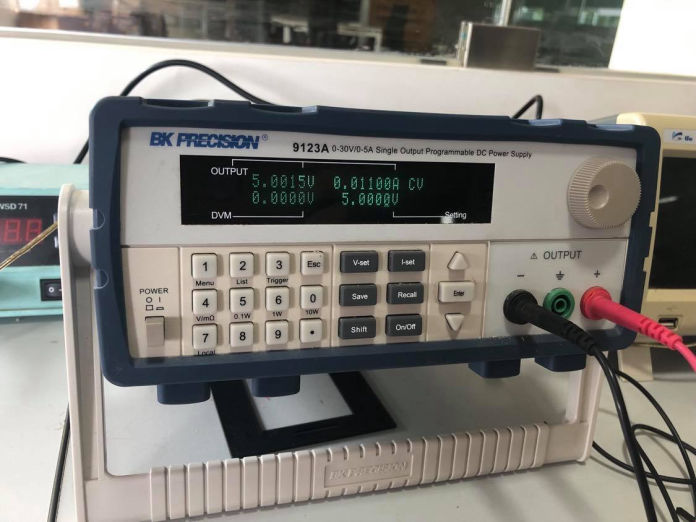Only Buzzer Connected ==> Voltage = 5V; Current = 0.05475A; Diff. in Current = 0.04375A; Power = V * I = 0.219W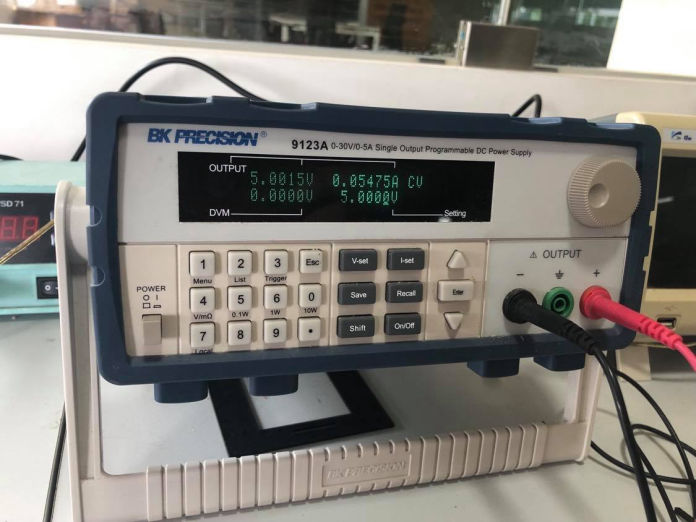Only Servo Connected ==> Voltage = 5V; Current = 0.71275A; Diff. in Current = 0.70175A; Power = V * I = 3.501W